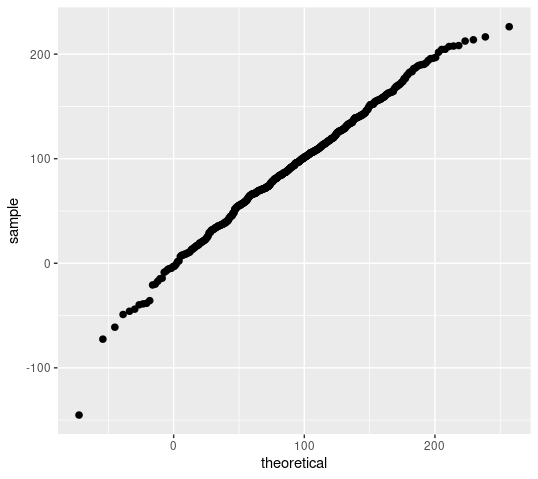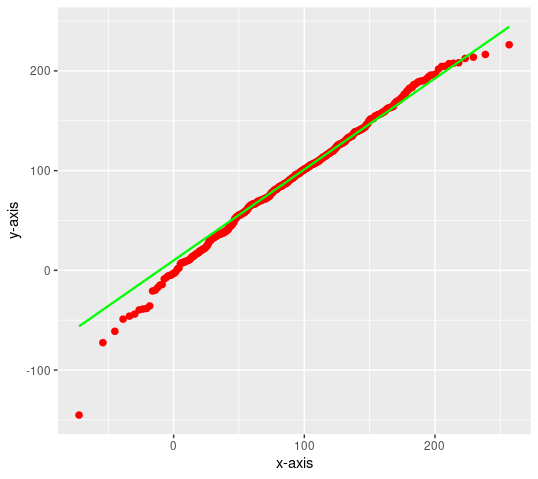Open in App
Not now

# Normal Probability Plot in R using ggplot2

• Last Updated : 14 Jan, 2022

A normal probability plot is a graphical representation of the data. A normal probability plot is used to check if the given data set is normally distributed or not. It is used to compare a data set with the normal distribution. If a given data set is normally distributed then it will reside in a shape like a straight line.

In this article, we are going to use ggplot2 with qqplotr to plot and check if the dataset is normally distributed using qqplot only.

### Approach

• Install the following necessary libraries by pasting them in r console

install.packages(“ggplot2”)

install.packages(“qqplotr”)

• Create a random data set with a different mean and standard deviation that you want to plot.
• Plotting data using stat_qq_point() method.
• Plotting data points with line using stat_qq_line() function.

Given below is a proper implementation using the above approach

Example 1: Plotting data using stat_qq_point() method.

## R

 `# importing libraries` `library``(ggplot2)` `library``(qqplotr)`   `# creating random data` `random_values = ``rnorm``(500, mean = 90, sd = 50)`   `# plotting data without line and labels` `ggplot``(mapping = ``aes``(sample = random_values)) + ``stat_qq_point``(size = 2)`

Output:Fig. 1 Plotting Data points.

Example 2: Plotting data points with line using stat_qq_line() function.

## R

 `# importing libraries` `library``(ggplot2)` `library``(qqplotr)`   `# creating random data` `random_values = ``rnorm``(500, mean = 90, sd = 50)`   `# plotting data with proper labels ` `# And adding line with proper properties` `ggplot``(mapping = ``aes``(sample = random_values)) ` `+ ``stat_qq_point``(size = 2,color = ``"red"``) ` `+ ``stat_qq_line``(color=``"green"``) ` `+ ``xlab``(``"x-axis"``) + ``ylab``(``"y-axis"``)`

Output: